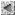## PhD Thesis

• PhD Thesis Title: Subdivision Schemes, Biorthogonal Wavelets and Image Compression
• Author: Bin Han
• Supervisor: Professor Rong-Qing Jia
• Location: Department of Mathematical Sciences, University of Alberta
• PhD thesis (total 138 pages and five chapters). An electronic copy is available from ProQuest LLC.

### Abstract of PhD Thesis for each chapter:

• In Chapter 1, we give a complete characterization of L_p convergence of any subdivision scheme associated with any general dilation matrix in arbitrary dimensions. We demonstrate that the L_2 convergence of any subdivision scheme can be easily checked by computing the spectral radius of a finite matrix.
• In Chapter 2, we give a sharp (optimal) error estimate in the L_p norm sense for any multivariate subdivision scheme when there is a perturbation in its mask. If the subdivision scheme associated with mask a converges in the L_p norm to \phi_a, then we demonstrate that if the mask b is close to the mask a, then the subdivision scheme associated with b converges to \phi_b in the L_p norm, and the difference between \phi_a and \phi_b in the L_p norm can be controlled by a constant C multiplying by the difference between the mask a and the mask b, where C depends only on a.
• In Chapter 3, based on a simple proof, we give a complete characterization of L_p smoothness of multivariate refinable functions with dilation matrix 2I_s. Then we demonstrate that if an inpterpolatory mask is supported on [1-2r, 2r-1]^s, then it can satisfy the sum rules of order at most 2r (sum rules of a mask are sort of approximation order or accuracy order of a function, and are closely related to vanishing moments). A function f is fundamental if f(0)=1 and f(j)=0 for all nonzero multi-integers j. We demonstrate that if \phi_a is a fundamental refinable function with an interpolatory mask a such that a is supported on [1-2r,2r-1]^s and satisfies the sum rules of order 2r, then its L_p smoothness described by the L_p critical exponent can not exceed the L_p smoothness of \phi_{b_r} where b_r is the unique Delauriers-Dubuc interpolatory mask supported on [1-2r,2r-1]. More remarkable is that a family of bivariate optimal interpolatory masks is given. Such interpolatory masks have full symmetry and achieve both the optimal order of sum rules and optimal L_2 smoothness order.
• In Chapter 4, the investigation about the optimal order of sum rules and optimal smoothness of interpolatory masks with respect to their support is carried out for the orthogonal and biorthogonal multivariate wavelets. More importantly, a construction by coset (CBC) algorithm is given to construct biorthogonal multivariate wavelets. Also a family of optimal bivariate biorthogonal wavelets is given. More specifically, for any interpolatory primal mask, such CBC algorithm can give us all the dual masks with arbitrary vanishing moments to the given primal mask. This CBC algorithm can be easily implemented and was generalized latter by my collaborators and myself to biorthogonal multiwavelets with any general dilation matrix in any dimension such that for any primal mask, it guarantees and gives us a dual mask of the given primal mask such that the dual mask has arbitrary vanishing moments. See my publication for more detail about CBC algorithm.
• In Chapter 5, we implemented a wavelet transform in C++ by directly using 2-D biorthogonal wavelet filters. Some experimental results are listed. The research in Chapter 5 is still in the phase of experiments and is still undergoing now.
• Note:. See my publication for detail about the above results

### Abstract of PhD Thesis of Bin Han

In this thesis, we study subdivision schemes, biorthogonal wavelets and wavelet-based image compression. Subdivision schemes are important in computer aided geometric design to generate curves and high dimensional surfaces. First, We shall characterize the $L_p$ convergence of any subdivision scheme with a finitely supported refinement mask in multivariate case. Then we shall study the error behavior of any subdivision scheme if there is a round-off of its refinement mask. Next, we study a special kind of subdivision schemes -- interpolatory subdivision schemes. We shall analyze the optimal properties, such as sum rules of an interpolatory refinement mask and the smoothness of its associated refinable function, of any interpolatory subdivision scheme. A general construction of optimal interpolatory subdivision schemes is presented. Next, we shall study biorthogonal multivariate wavelets since there is a well known close relation between interpolatory subdivision schemes and biorthogonal wavelets. We shall study the optimal approximation and smoothness properties of any biorthogonal wavelet. More importantly, a general and easy way (CBC algorithm) is presented to construct multivariate biorthogonal wavelets. As an example, a family of optimal bivariate biorthogonal wavelets is given. Finally, we try to apply our results in image compression and a 2-D wavelet transform C++ program is established which can use a library of bivariate biorthogonal wavelet filters.Back to Research Home Page